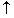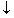# The Algebra Underlying Account Rules:A Teaching Note

David O’Bryan, Ph.D., CPA, CMA, CFM, CFE

Becky Casey, MBA, CPA

Cynthia Woodburn, Ph.D.

Abstract

The purpose of this teaching note is to provide a formal linkage between the algebra of the accounting equation and the double entry system utilizing debit and credit rules.  Instructors may find this material useful in helping their students understand the logical and mathematical foundation for the debit and credit rules.  This material is especially helpful for students who want to understand “why” – not just “how.”

The Algebra Underlying Account Rules:  A Teaching Note:

David O’Bryan, Ph.D., CPA, CMA, CFM, CFE*

Associate Professor and Chairperson

Department of Accounting, Kelce College of Business

Pittsburg State University, Pittsburg, Kansas  66762

Email: obryan@pittstate.edu  Voice: (620) 235-4566  Fax: (620) 235-4558

Becky Casey, MBA, CPA

Instructor

Pittsburg State University

Cynthia Woodburn, Ph.D.

Associate Professor

Pittsburg State University

*Contact Author

One of the most awkward points in teaching financial or intermediate accounting is transitioning to the double entry system utilizing debit and credit rules.  User oriented financial accounting textbooks commonly use an algebraic approach by recording increases and decreases with pluses and minuses.  At some point, if not in financial accounting, certainly in intermediate accounting, the instructor needs to transition to the debit and credit approach used by practitioners.

For an instructor, this can be frustrating.  Some students who were doing very well using the algebraic approach become quite confused by the debit and credit approach.  These students fail to see the equivalency of the two approaches.  Instead, they view the debit and credit approach as an entirely new method of recording transactions.

This should not be surprising.  The equivalency of the two approaches is not transparent.  Textbooks generally begin with the accounting equation, followed by the t-accounts and debit and credit rules.  A formal and explicit linkage between the accounting equation and the debit and credit rules is not found in any published introductory or intermediate financial accounting textbook.  In the absence of a formal connection, students memorize account rules but may fail to realize the underlying reason for them.

The purpose of this short teaching note is to provide instructors a concise linkage between the accounting equation and the debit and credit account rules.  In four short steps an instructor can demonstrate to students that the debit and credit account rules are a direct derivation of the algebra of the accounting equation.  This material provides an instructor with a mathematical foundation for the account rules rather than the ad hoc, leap-of-faith approach provided in textbooks.  In addition, students can use the approach to derive the account rules rather than memorize them.  For instructors who stress understanding over memorization, this approach may be attractive.

# The Teaching Approach

This section describes a method to link the accounting equation to the debit and credit account rules.  First, start with the basic accounting equation:

+ A = + L + E                                           (1)

where A = assets, L = liabilities, and E = stockholders’ equity.

Second, allow for either an increase or a decrease in each element:

+A -A = + L -L +E -E                     (2)

Third, rearrange the terms by putting all terms to the left of the equals sign.  From basic algebra, remember to switch the sign of elements being moved from the right to the left side.  Arrange the terms so that the positive-signed component of each element is listed first followed by its respective negative-signed component:

+A -A +L -L + E -E = 0                     (3)

Now, visualize a “t-account” beneath each element of the accounting equation, with the positive-signed component appearing on the left-hand side of the t-account and the negative-signed component appearing in the right-hand side of the t-account:The mathematical connection between the accounting equation and the double-entry system is now apparent from equation (4).  The left-hand, or debit, side of the t-account is
simply for the terms with positive signs in equation (4).  Likewise, the right-hand, or credit, side of the t-account is for the terms with negative signs in equation (4).

An alternative approach is to begin with equation (3) and group like-signed terms together.  Then visualize two columns beneath this equation; a column for the positive-signed terms and a column for the negative-signed terms:Equation (5) complements equation (4) and reinforces the concept that the algebraic sign of each term determines whether it is a debit or credit.  That is, the account rules flow directly from the underlying algebra of the accounting equation.

# Conclusion

This teaching approach may be attractive to instructors who want their students to understand the logic of the account rules.  In fact, it is an open question how many practitioners truly understand the mathematical foundation for the debit and credit rules.  Students and practitioners can no doubt survive and prosper without understanding this linkage.  However, the account rules are so fundamental to our profession that it seems as though we would want to understand “why” – not just “how.”